Courses

# Test: Entropy - 1

## 10 Questions MCQ Test Topicwise Question Bank for Mechanical Engineering | Test: Entropy - 1

Description
This mock test of Test: Entropy - 1 for Mechanical Engineering helps you for every Mechanical Engineering entrance exam. This contains 10 Multiple Choice Questions for Mechanical Engineering Test: Entropy - 1 (mcq) to study with solutions a complete question bank. The solved questions answers in this Test: Entropy - 1 quiz give you a good mix of easy questions and tough questions. Mechanical Engineering students definitely take this Test: Entropy - 1 exercise for a better result in the exam. You can find other Test: Entropy - 1 extra questions, long questions & short questions for Mechanical Engineering on EduRev as well by searching above.
QUESTION: 1

### A body of constant heat capacity Cp and Initial temperature T1 is placed in contact with a heat reservoir at temperature T2 and comes to isothermal equilibrium with it. If T2 > T1 what is the entropy change of the universe?

Solution: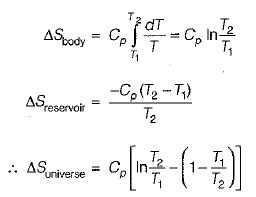QUESTION: 2

### When a system is in equilibrium, any conceivable change in entropy would be

Solution: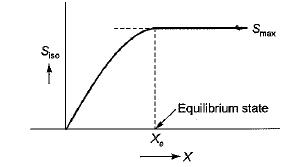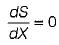at the peak of the entropy hill.

QUESTION: 3

### Match List-I (Laws of thermodynamics) with List-II (Defines) the following: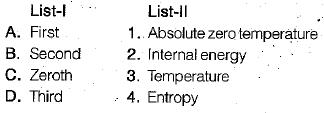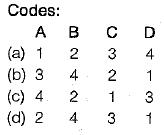Solution:
QUESTION: 4

Consider the processes:

Process A: A heat source at 800 K loses 2000 kJ of heat to sink at 500 K.
Process B: A heat source at 800 K loses 2000 kJ of heat to sink at 750 K.
Which of the following can be concluded?

Solution: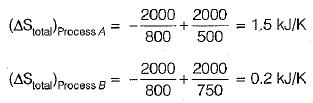Thus, total entropy change for the process B is smaller and therefore it is less irreversible.

QUESTION: 5

A piston-cylinder device contains a liquid-vapor mixture of water at 300 K. During a constant- pressure process, 750 kJ of heat is transferred to the water. As a result, part of the liquid in the cylinder vaporizes. What is entropy change of the water during this process?

Solution:

The system undergoes an internally reversible, isothermal process, and thus its entropy change,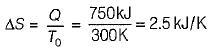QUESTION: 6

The slope of constant pressure line on a T-s diagram is given by

Solution:

TdS= dH - VdP
TdS = Cp dT - VdP
TdS - Cp d T - 0
(for constant pressure process)

∴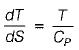QUESTION: 7

The slope of constant volume line on a T-s diagram is given by

Solution: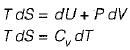(for constant volume process)
∴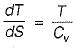QUESTION: 8

The value of entropy at 0 K is taken as

Solution:

S = K ln W
where, S is entropy
K is Boltzmann constant
W is thermodynamic probability
when W = 1, it represents the greatest order,
S = 0. This occurs only at T = 0 K. This is the Nernst-Simon statement of third law of themnodyanamics.

QUESTION: 9

Choose the correct statement:

Solution:
QUESTION: 10

Which of the following second-law statements is incorrect?

Solution: